Properties of Determinant

Chapter 4 Class 12 Determinants
Serial order wise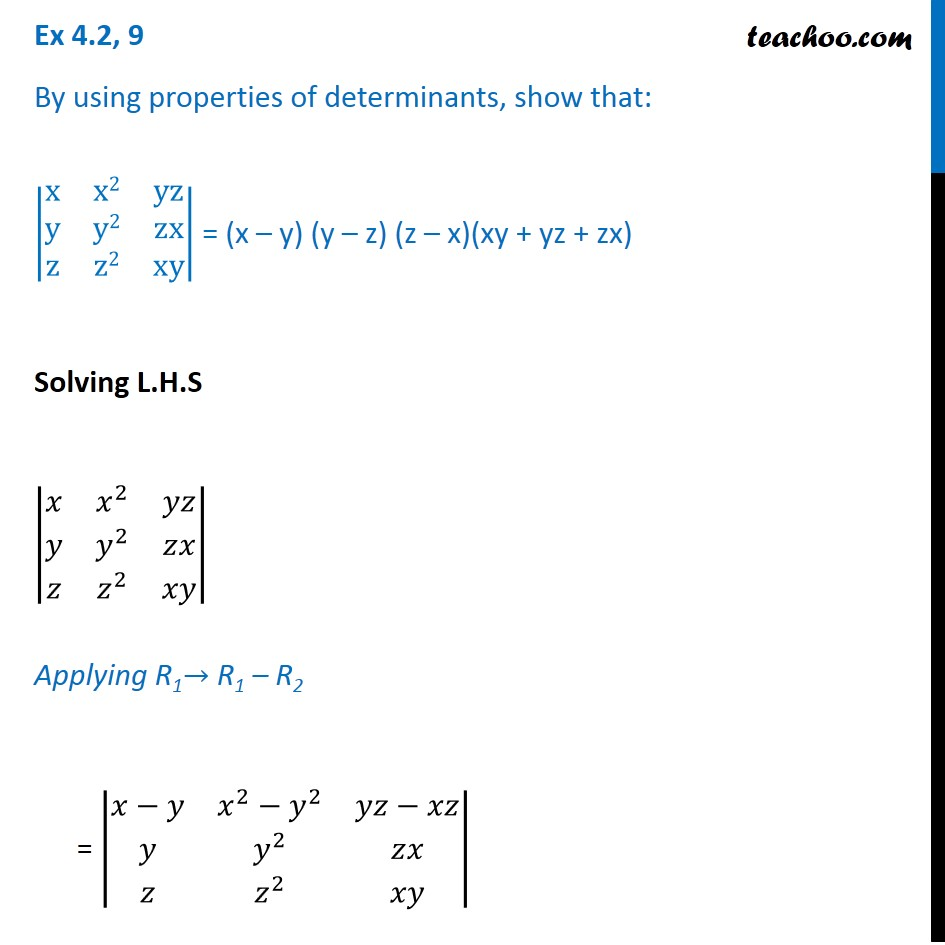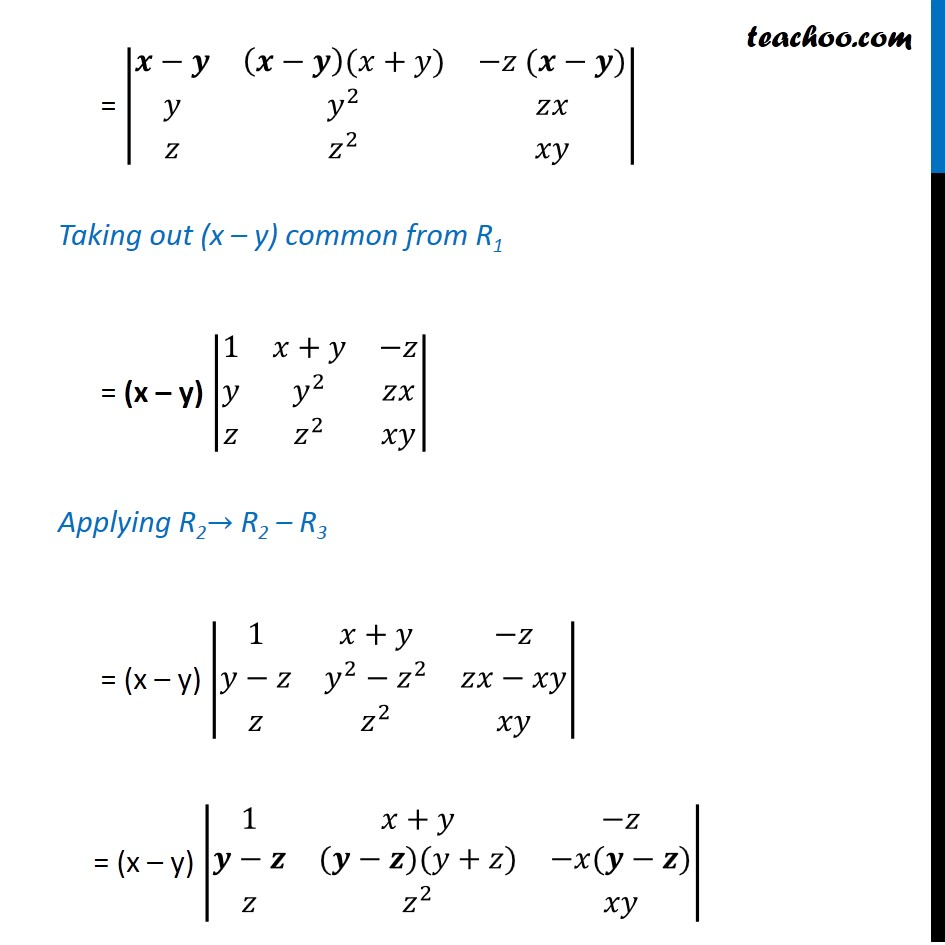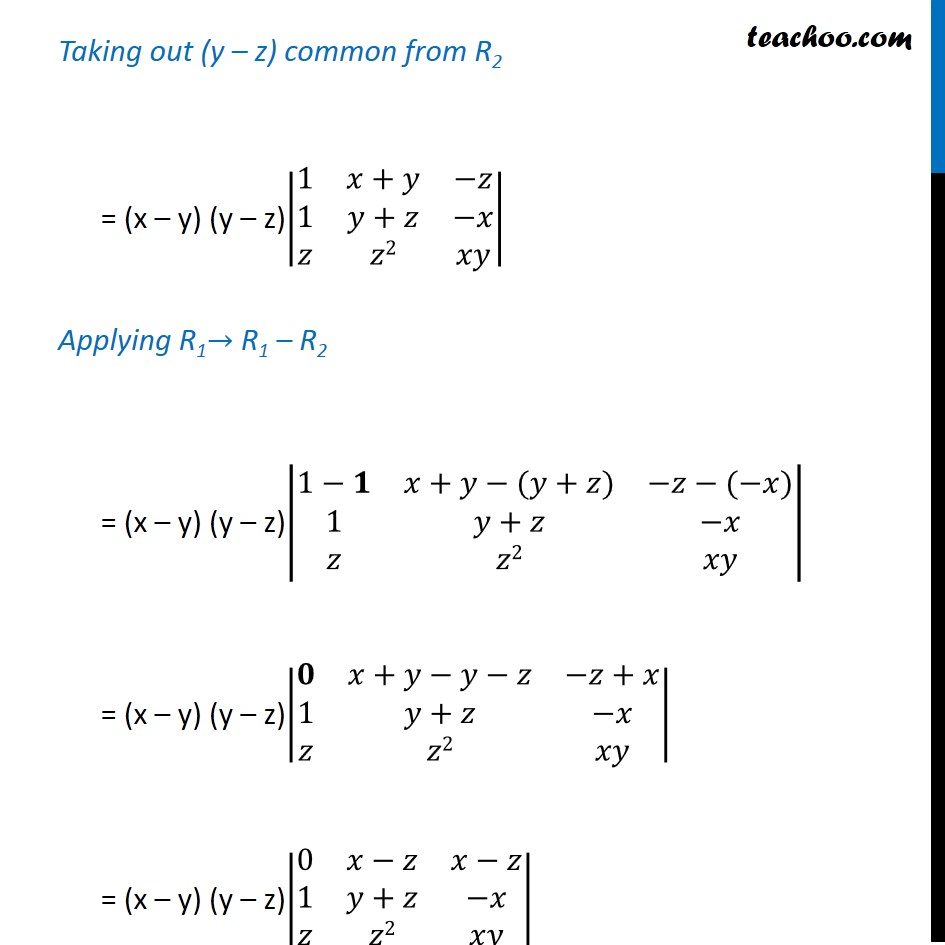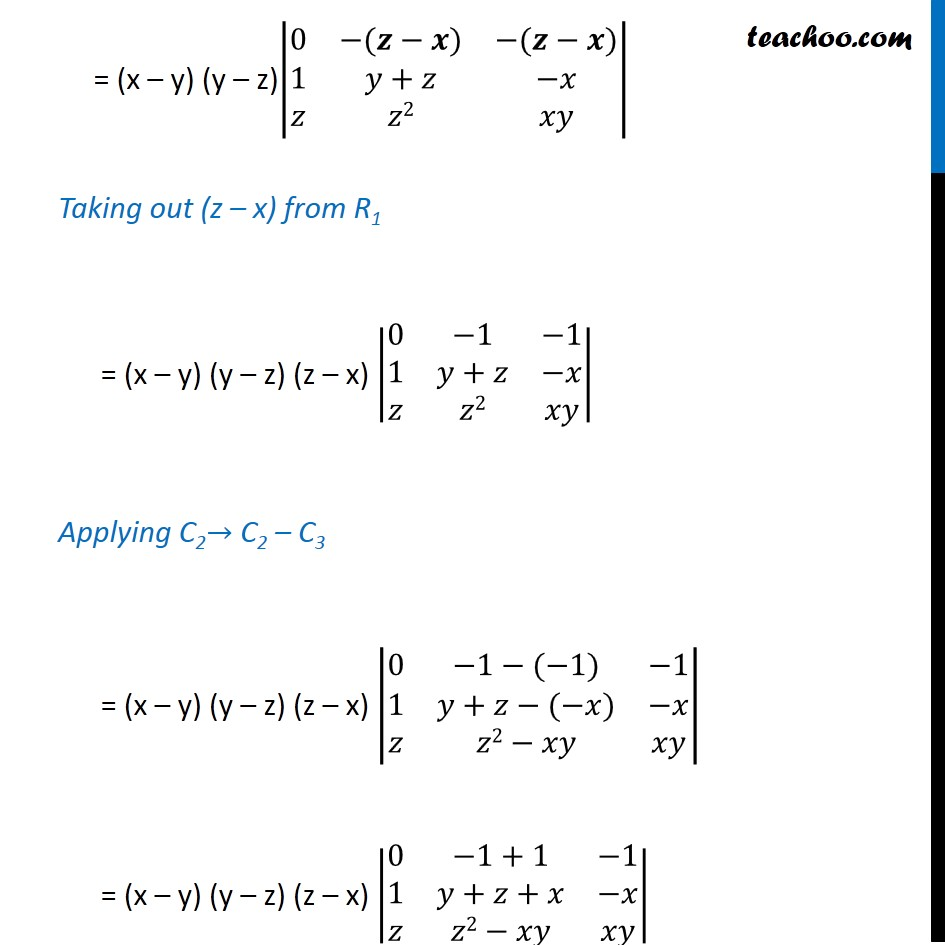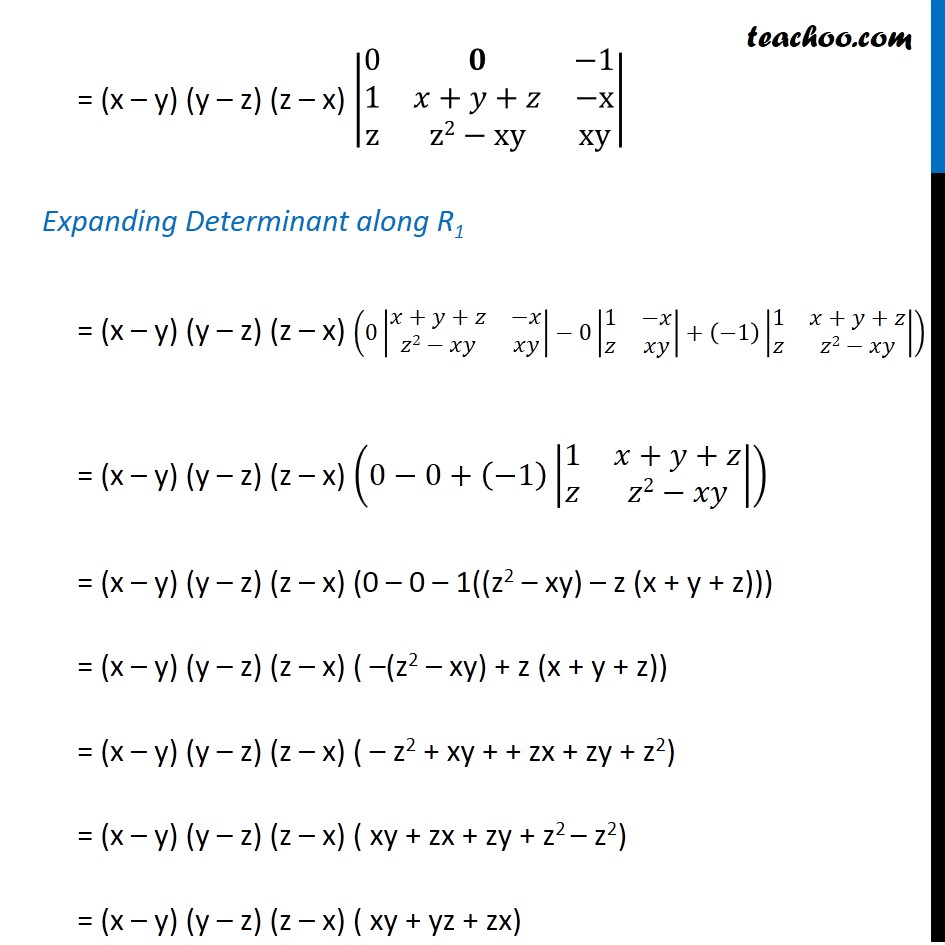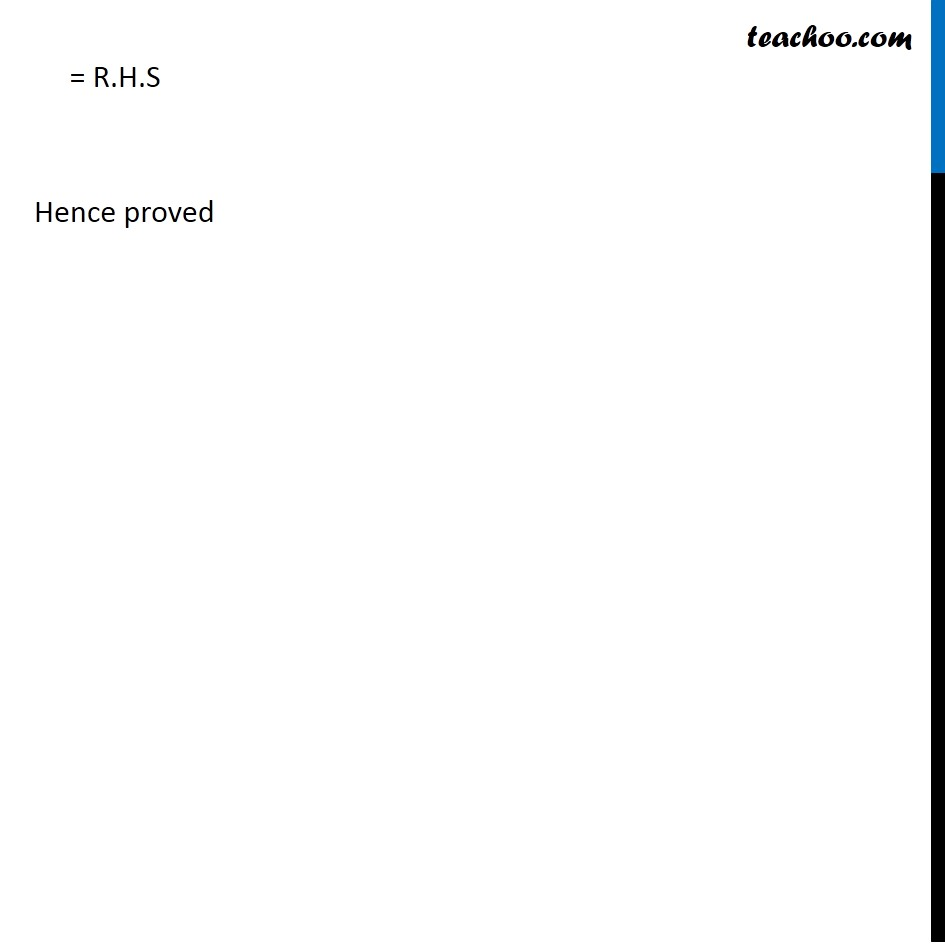Learn in your speed, with individual attention - Teachoo Maths 1-on-1 Class

### Transcript

Question 9 By using properties of determinants, show that: |■8(x&x2&yz@y&y2&zx@z&z2&xy)| = (x – y) (y – z) (z – x)(xy + yz + zx) Solving L.H.S |■8(𝑥&𝑥^2&𝑦𝑧@𝑦&𝑦^2&𝑧𝑥@𝑧&𝑧^2&𝑥𝑦)| Applying R1→ R1 – R2 = |■8(𝑥−𝑦&𝑥^2−𝑦^2&𝑦𝑧−𝑥𝑧@𝑦&𝑦^2&𝑧𝑥@𝑧&𝑧^2&𝑥𝑦)| Question 9 By using properties of determinants, show that: |■8(x&x2&yz@y&y2&zx@z&z2&xy)| = (x – y) (y – z) (z – x)(xy + yz + zx) Solving L.H.S |■8(𝑥&𝑥^2&𝑦𝑧@𝑦&𝑦^2&𝑧𝑥@𝑧&𝑧^2&𝑥𝑦)| Applying R1→ R1 – R2 = |■8(𝑥−𝑦&𝑥^2−𝑦^2&𝑦𝑧−𝑥𝑧@𝑦&𝑦^2&𝑧𝑥@𝑧&𝑧^2&𝑥𝑦)| = |■8(𝒙−𝒚&(𝒙−𝒚)(𝑥+𝑦)&−𝑧 (𝒙−𝒚)@𝑦&𝑦^2&𝑧𝑥@𝑧&𝑧^2&𝑥𝑦)| Taking out (x – y) common from R1 = (x – y) |■8(1&𝑥+𝑦&−𝑧@𝑦&𝑦^2&𝑧𝑥@𝑧&𝑧^2&𝑥𝑦)| Applying R2→ R2 – R3 = (x – y) |■8(1&𝑥+𝑦&−𝑧@𝑦−𝑧&𝑦^2−𝑧^2&𝑧𝑥−𝑥𝑦@𝑧&𝑧^2&𝑥𝑦)| = (x – y) |■8(1&𝑥+𝑦&−𝑧@𝒚−𝒛&(𝒚−𝒛)(𝑦+𝑧)&−𝑥(𝒚−𝒛)@𝑧&𝑧^2&𝑥𝑦)| Taking out (y – z) common from R2 = (x – y) (y – z)|■8(1&𝑥+𝑦&−𝑧@1&𝑦+𝑧&−𝑥@𝑧&𝑧2&𝑥𝑦)| Applying R1→ R1 – R2 = (x – y) (y – z)|■8(1−𝟏&𝑥+𝑦−(𝑦+𝑧)&−𝑧−(−𝑥)@1&𝑦+𝑧&−𝑥@𝑧&𝑧2&𝑥𝑦)| = (x – y) (y – z)|■8(𝟎&𝑥+𝑦−𝑦−𝑧&−𝑧+𝑥@1&𝑦+𝑧&−𝑥@𝑧&𝑧2&𝑥𝑦)| = (x – y) (y – z)|■8(0&𝑥−𝑧&𝑥−𝑧@1&𝑦+𝑧&−𝑥@𝑧&𝑧2&𝑥𝑦)| = (x – y) (y – z)|■8(0&−(𝒛−𝒙)&−(𝒛−𝒙)@1&𝑦+𝑧&−𝑥@𝑧&𝑧2&𝑥𝑦)| Taking out (z – x) from R1 = (x – y) (y – z) (z – x) |■8(0&−1&−1@1&𝑦+𝑧&−𝑥@𝑧&𝑧2&𝑥𝑦)| Applying C2→ C2 – C3 = (x – y) (y – z) (z – x) |■8(0&−1−(−1)&−1@1&𝑦+𝑧−(−𝑥)&−𝑥@𝑧&𝑧2−𝑥𝑦&𝑥𝑦)| = (x – y) (y – z) (z – x) |■8(0&−1+1&−1@1&𝑦+𝑧+𝑥&−𝑥@𝑧&𝑧2−𝑥𝑦&𝑥𝑦)| = (x – y) (y – z) (z – x) |■8(0&𝟎&−1@1&𝑥+𝑦+𝑧&−x@z&z2−xy&xy)| Expanding Determinant along R1 = (x – y) (y – z) (z – x) (0|■8(𝑥+𝑦+𝑧&−𝑥@𝑧2−𝑥𝑦&𝑥𝑦)|−0|■8(1&−𝑥@𝑧&𝑥𝑦)|+(−1)|■8(1&𝑥+𝑦+𝑧@𝑧&𝑧2−𝑥𝑦)|) = (x – y) (y – z) (z – x) (0−0+(−1)|■8(1&𝑥+𝑦+𝑧@𝑧&𝑧2−𝑥𝑦)|) = (x – y) (y – z) (z – x) (0 – 0 – 1((z2 – xy) – z (x + y + z))) = (x – y) (y – z) (z – x) ( –(z2 – xy) + z (x + y + z)) = (x – y) (y – z) (z – x) ( – z2 + xy + + zx + zy + z2) = (x – y) (y – z) (z – x) ( xy + zx + zy + z2 – z2) = (x – y) (y – z) (z – x) ( xy + yz + zx) = R.H.S Hence proved